# Lesson 5. Calculate and Plot Difference Normalized Burn Ratio (dNBR) from Landsat Remote Sensing Data in R

## Learning Objectives

After completing this tutorial, you will be able to:

• Calculate dNBR in R with Landsat data.

## What You Need

You will need a computer with internet access to complete this lesson and the data for week 8 of the course.

As discussed in the previous lesson, you can use dNBR to map the extent and severity of a fire. In this lesson, you learn how to create NBR using Landsat data.

You calculate dNBR using the following steps:

1. Open up pre-fire data and calculate NBR
2. Open up the post-fire data and calculate NBR
3. Calculate dNBR (difference NBR) by subtracting post-fire NBR from pre-fire NBR (NBR pre - NBR post fire).
4. Classify the dNBR raster using the classification table provided below and isn the previous lesson.

Note the code to do this is hidden. You will need to figure out what bands are required to calculate NBR using Landsat.

## Calculate dNBR Using Landsat Data

First, let’s setup your spatial packages.

# load spatial packages
library(raster)
library(rgdal)
library(rgeos)
library(RColorBrewer)
library(dplyr)

# turn off factors
options(stringsAsFactors = FALSE)

# source the normalized diff function that you write for week_7
#source("ea-course-functions.R")


Next, open up the pre- Cold Springs fire Landsat data. Create a rasterbrick from the bands. Then calculate NBR. A plot of NBR is below.

all_landsat_bands_post <- list.files("data/week-08/landsat/LC80340322016205-SC20170127160728/crop",
pattern = glob2rx("*band*.tif\$"),
full.names = TRUE) # use the dollar sign at the end to get all files that END WITH
all_landsat_bands_post
##  "data/week-08/landsat/LC80340322016205-SC20170127160728/crop/LC80340322016205LGN00_sr_band1_crop.tif"
##  "data/week-08/landsat/LC80340322016205-SC20170127160728/crop/LC80340322016205LGN00_sr_band2_crop.tif"
##  "data/week-08/landsat/LC80340322016205-SC20170127160728/crop/LC80340322016205LGN00_sr_band3_crop.tif"
##  "data/week-08/landsat/LC80340322016205-SC20170127160728/crop/LC80340322016205LGN00_sr_band4_crop.tif"
##  "data/week-08/landsat/LC80340322016205-SC20170127160728/crop/LC80340322016205LGN00_sr_band5_crop.tif"
##  "data/week-08/landsat/LC80340322016205-SC20170127160728/crop/LC80340322016205LGN00_sr_band6_crop.tif"
##  "data/week-08/landsat/LC80340322016205-SC20170127160728/crop/LC80340322016205LGN00_sr_band7_crop.tif"

# stack the data
landsat_post_st <- stack(all_landsat_bands_post)
landsat_post_br <- brick(landsat_post_st)


# this code shows you how to create a normalized Z value for your raster plots!
# it also uses color brewer to color the output raster
# display.brewer.all()
nbr_colors <- colorRampPalette(brewer.pal(11, "PiYG"))(100)

plot(landsat_nbr_prefire,
main = "Landsat derived NBR\n Pre-Fire \n with fire boundary overlay",
axes = FALSE,
box = FALSE,
col = nbr_colors,
zlim = c(-1, 1))
plot(fire_boundary_utm,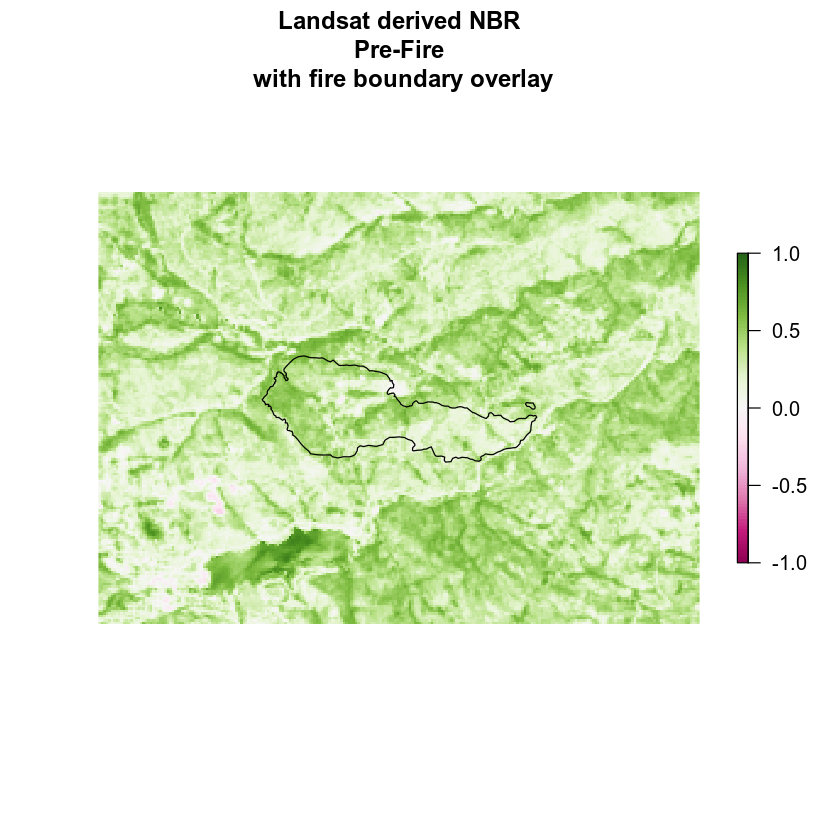You can export the NBR raster if you want using writeRaster().

check_create_dir("data/week-08/outputs/landsat_nbr")
writeRaster(x = landsat_nbr_prefire,
filename="data/week-08/outputs/landsat_nbr",
format = "GTiff", # save as a tif
datatype='INT2S', # save as a INTEGER rather than a float
overwrite = TRUE)


Next, open the post-fire landsat data to calculate post-fire NBR. If you want to use the post-fire data to CROP the pre fire data you may do this in a different order.

Then you calculate NBR on the post data - note the code here is purposefully hidden. You need to figure out what bands to use to perform the math!

# notice that the data are plotting using the same z limits to fix the color ramp
plot(landsat_nbr_postfire,
main = "Landsat derived Normalized Burn Index (NBR)\n Post Fire",
axes = FALSE,
box = FALSE,
col = nbr_colors,
zlim = c(-1, 1))
plot(fire_boundary_utm,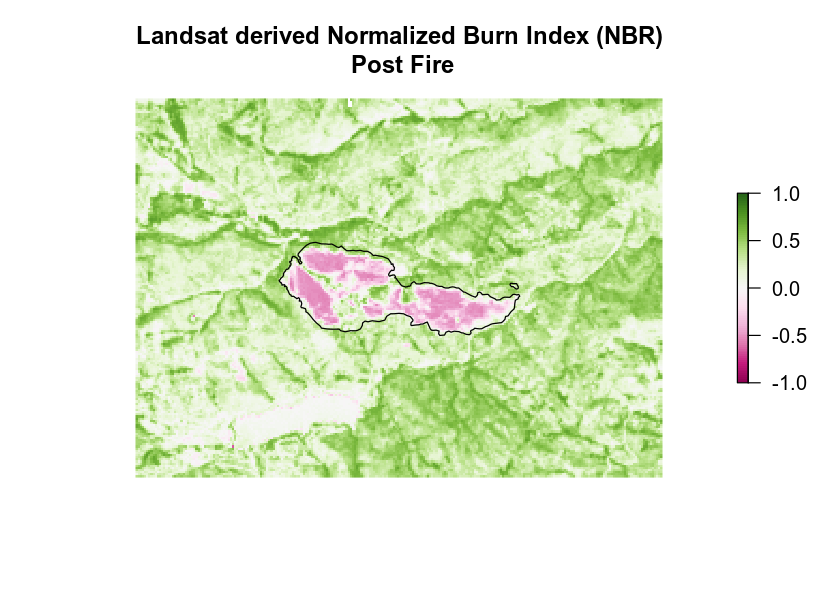Finally, calculate the DIFFERENCE between the pre and post NBR.

# calculate difference
landsat_nbr_diff <- landsat_nbr_prefire - landsat_nbr_postfire
plot(landsat_nbr_diff,
main = "Difference NBR map \n Pre minus post Cold Springs fire",
axes = FALSE, box = FALSE)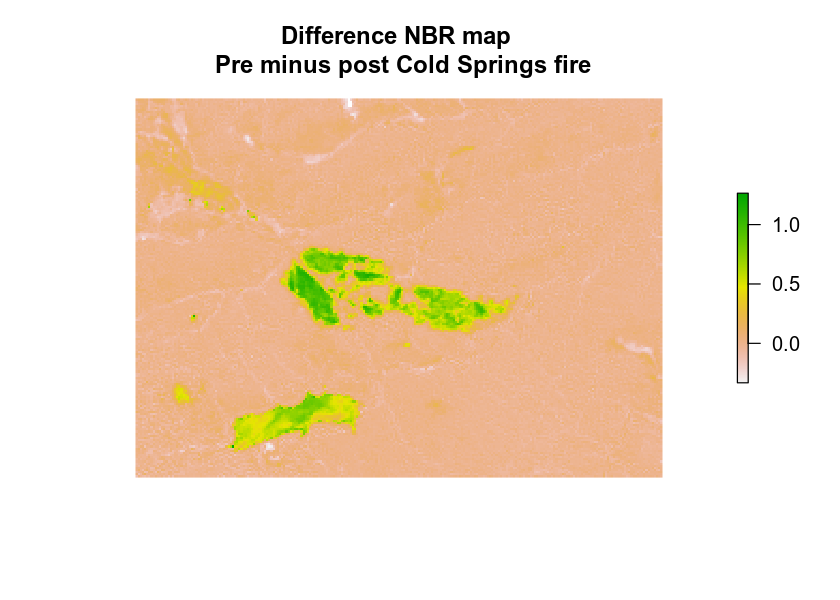When you have calculated dNBR or the difference in NBR pre minus post fire, classify the output raster using the classify() function and the classes below. You learned how to classify rasters in the Earth Analytics lidar lessons

SEVERITY LEVEL dNBR RANGE
Enhanced Regrowth > -.1
Unburned -.1 to +.1
Low Severity +.1 to +.27
Moderate Severity +.27 to +.66
High Severity > +1.3

NOTE: your min an max values for NBR may be slightly different from the table shown above! If you have a smaller min value (< -700) then adjust your first class to that smallest number. If you have a largest max value (>1300) then adjust your last class to that largest value in your data.

Alternatively, you can use the Inf to specify the smallest -Inf and largest Inf values.

You classified map should look something like: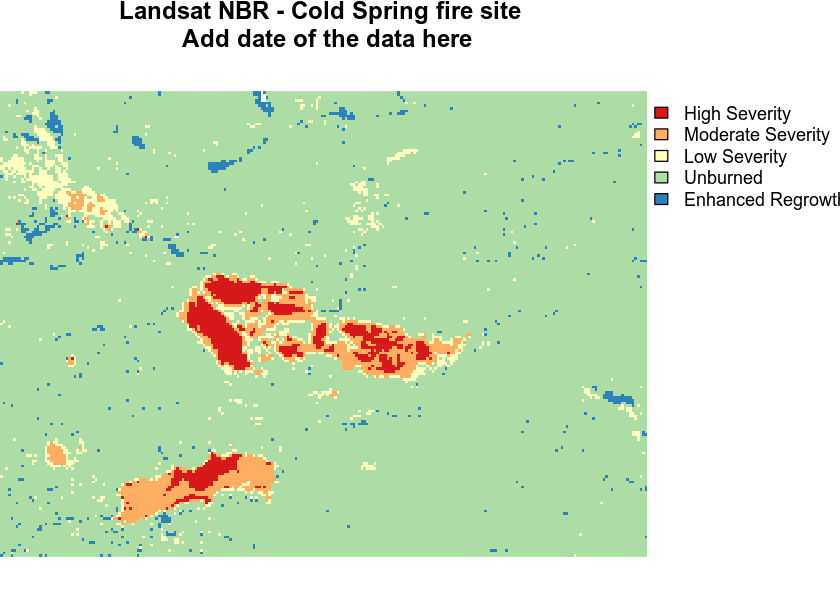## Compare to Fire Boundary

As an example to see how your fire boundary relates to the boundary that you’ve identified using MODIS data, you can create a map with both layers. I’m using the shapefile in the folder:

data/week-08/vector_layers/fire-boundary-geomac/co_cold_springs_20160711_2200_dd83.shp

Add fire boundary to map. Note the “Spectral” colorbrewer ramp is used in the map below.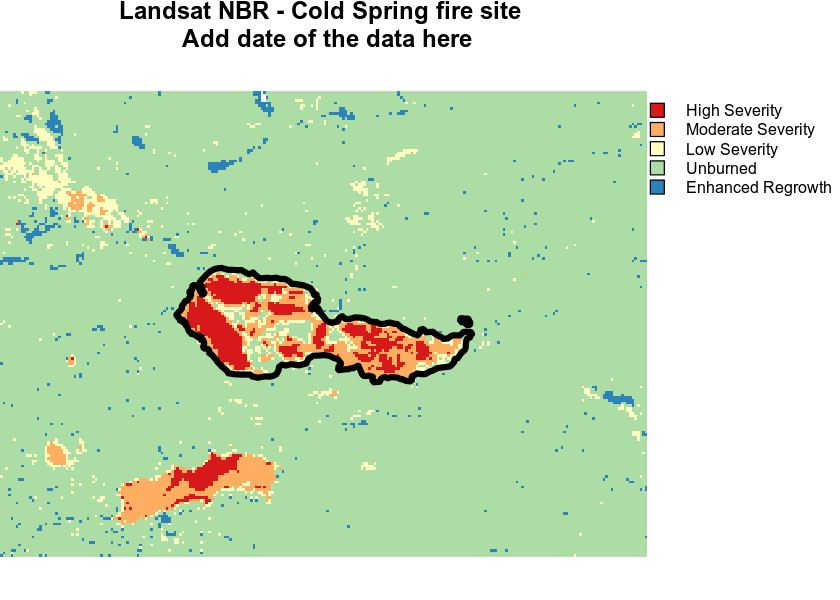Make it look a bit nicer using a colorbrewer palette. I used the RdYlGn palette:

brewer.pal(5, 'RdYlGn')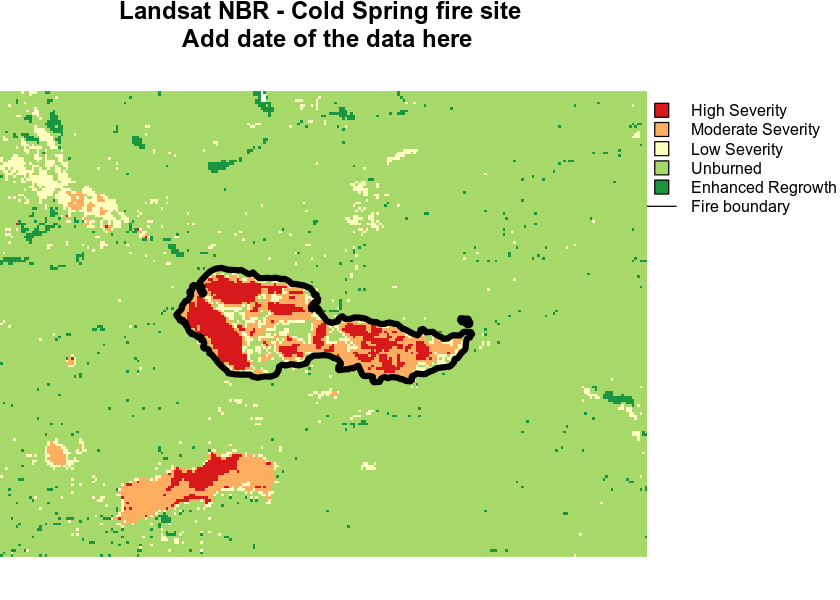Note that you will have to figure out what date these data are for! I purposefully didn’t include it in the title of this map.

barplot(nbr_classified,
main = "Distribution of Classified NBR Values",
col = the_colors,
names.arg = c("Enhanced \nRegrowth", "Unburned", "Low \n Severity", "Moderate \n Severity", "High \nSeverity"))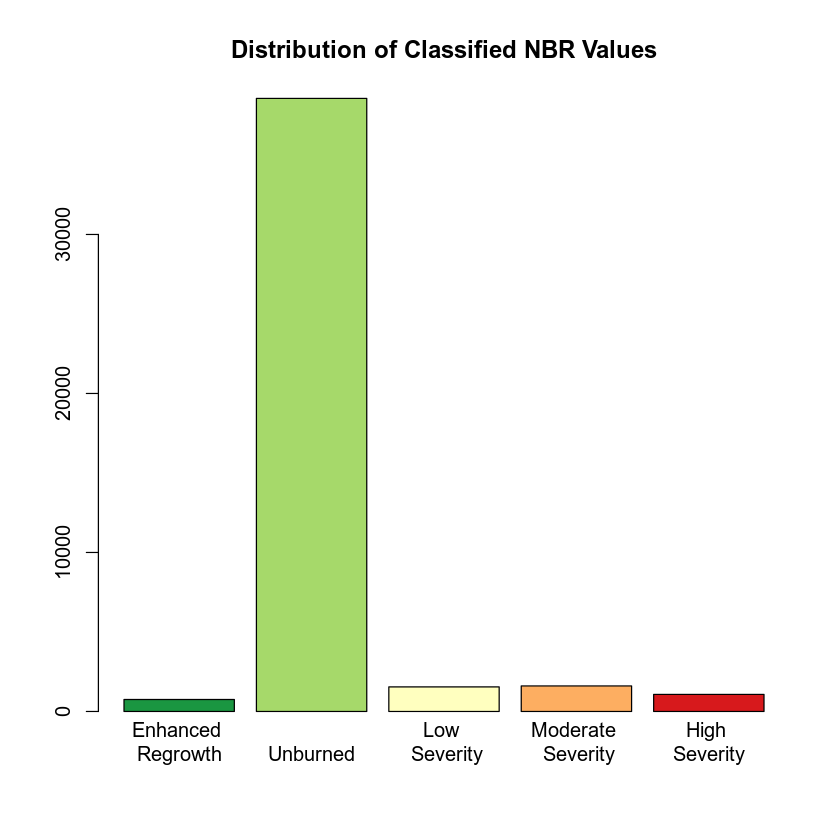## Calculate Total Area of Burned Area

Once you have classified your data, you can calculate the total burn area.

To calculate this you could either

1. use the extract() function to extract pixels within the burn area boundary
2. crop() the data to the burn area boundary extent. This is an ok option however you have pixels represented that are outside of the burn boundary.
landsat_pixels_in_fire_boundary <- raster::extract(nbr_classified, fire_boundary_utm,
df = TRUE)

landsat_pixels_in_fire_boundary %>%
group_by(layer) %>%
summarize(count = n(), area_meters = n() * (30 * 30))